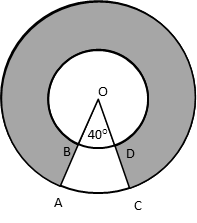# CBSE Class 10 Math Question Paper 2016 | Q14

###### CBSE Maths Board Paper Solution | 3 Mark | Geometry: Areas Related to CirclesClass 10 Maths Questions

The given question is a easy 3-mark question from the chapter Areas Related to Circles. Computing area of two concentric sectors is the concept tested in this question. It is an important question and appeared in Section C of the 2016 CBSE class 10 board paper.

Question 14: Find the area of the shaded region, enclosed between two concentric circles of radii 7 cm and 14 cm where ∠AOC = 40°. (Use π = $$frac{22}{7}$)## Target Centum in CBSE 10th Maths #### Free Online CBSE Coaching online.maxtute.com ### Video Explanation ## NCERT Solution to Class 10 Maths #### With Videos ### Explanatory Answer | CBSE 2016 Maths Paper Q14Area of the circular disc = Area of outer circle - Area of inner circle Radius of outer circle = 14 cm Radius of inner circle = 7 cm Area of the circular disc = π$14)2 - π(7)2

However, the shaded region does not include all 360°. It subtends an angle of 320°
∴ Area of shaded region = $$frac{320}{360}${π$14)2 - π(7)2}
= $$frac{8}{9}$ × $\frac{22}{7}$ ×$142 - 72)
= $$frac{8}{9}$ × $\frac{22}{7}$ ×$14 + 7) × (14 - 7)
= $\frac{8}{9}$ × $\frac{22}{7}$ × 21 × 7 = $\frac{8}{9}$ × 22 ×21
= $\frac{1232}{3}$sqcm.

###### Try CBSE Online CoachingClass 10 Maths

Register in 2 easy steps and
Start learning in 5 minutes!